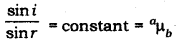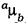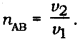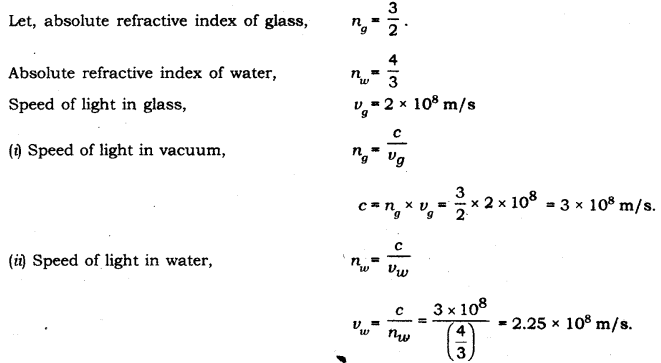# State the law of refraction of light that defines the refractive index of a medium with respect to the other

State the law of refraction of light that defines the refractive index of a medium with respect to the other. Express it mathematically. How is refractive index of any medium ‘A’ with respect to a medium ‘B’ related to the speed of propagation of light in two media A and B? State the name of this constant when one medium is vacuum or air. The refractive indices of glass and water with respect to vacuum are 3/2 and 4/3 respectively. If the speed of light in glass is 2 x 108 m/s. find the speed of light in
(i) vacuum,
(ii) water.

The law of refraction that defines the refractive index of a medium with respect to the other is given by first law of refraction known as Snell’s law.

First law of refraction:
The ratio of the sine of the angle of incidence to the sine of the angle of refraction is constant. This is known as Snell’s law. Mathematically, it can be given as follows:Here,is the relative refractive index of medium b with respect to medium a. Consider a ray of light travelling from medium B into medium A. Let v1 be the speed of light in medium A and v2 be the speed of light in medium B. Then the refractive index of medium

A with respect to medium B is given byIf one medium is vacuum or air then the constant is named as absolute refractive index of the medium.